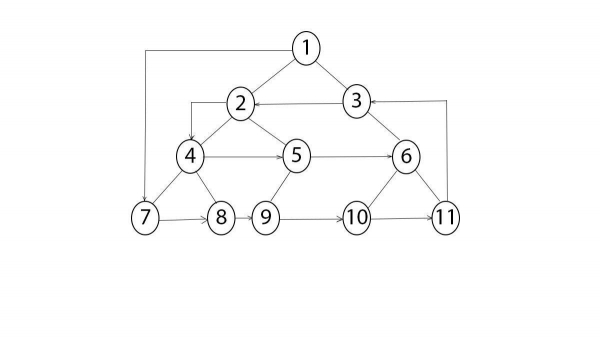# Anti Clockwise spiral traversal of a binary tree in C++?

C++Server Side ProgrammingProgramming

Anti-Clockwise spiral traversal of a binary tree is traversing the elements of a tree in such a way that if traversed they make a spiral but in reverse order. The following figure shows how a anti-clockwise spiral traversal of binary tree.The Algorithm defined for spiral traversal of a binary tree works in the following way −

Two variables i and j are initialized and values are equated as i = 0 and j = height of variable. A flag is used to check while section is to be printed. Flag is initially is set of false. A loop working till i < j, prints first half otherwise prints the last one and flips the flag value. This happens until the whole binary tree is printed.

## Example

Live Demo

#include <bits/stdc++.h>
using namespace std;
struct Node {
struct Node* left;
struct Node* right;
int data;
Node(int data) {
this->data = data;
this->left = NULL;
this->right = NULL;
}
};
int height(struct Node* root) {
if (root == NULL)
return 0;
int lheight = height(root->left);
int rheight = height(root->right);
return max(1 + lheight, 1 + rheight);
}
void leftToRight(struct Node* root, int level) {
if (root == NULL)
return;
if (level == 1)
cout << root->data << " ";
else if (level > 1) {
leftToRight(root->left, level - 1);
leftToRight(root->right, level - 1);
}
}
void rightToLeft(struct Node* root, int level) {
if (root == NULL)
return;
if (level == 1)
cout << root->data << " ";
else if (level > 1) {
rightToLeft(root->right, level - 1);
rightToLeft(root->left, level - 1);
}
}
int main() {
struct Node* root = new Node(1);
root->left = new Node(2);
root->right = new Node(3);
root->left->left = new Node(4);
root->right->left = new Node(5);
root->right->right = new Node(7);
root->left->left->left = new Node(10);
root->left->left->right = new Node(11);
root->right->right->left = new Node(8);
int i = 1;
int j = height(root);
int flag = 0;
while (i <= j) {
if (flag == 0) {
rightToLeft(root, i);
flag = 1;
i++;
} else {
leftToRight(root, j);
flag = 0;
j--;
}
}
return 0;
}

## Output

1 10 11 8 3 2 4 5 7